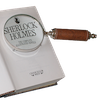#### You may also like### Number Detective

Follow the clues to find the mystery number.### Six Is the Sum

What do the digits in the number fifteen add up to? How many other numbers have digits with the same total but no zeros?### ABC

In the multiplication calculation, some of the digits have been replaced by letters and others by asterisks. Can you reconstruct the original multiplication?

# Spell by Numbers

##### Age 7 to 11Challenge Level

Here are some clues to help if you are stuck...

• $A, E, H$ and $T$ are evenly divisible by $2$
• $G, I, P$ and $U$ are not evenly divisible by $2$
• $T$ and $U$ are square numbers
• $T$ has twice the value $A$ but only half the value of $H$
• $P$ is only half of $E$ but three times $S$
• $G$ is greater than $I$